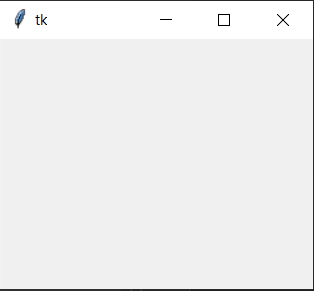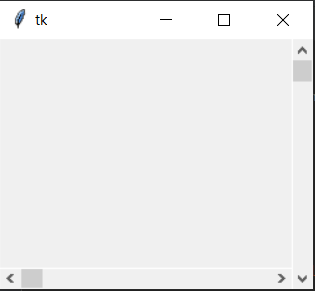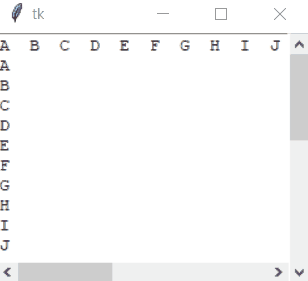# How to make a proper double scrollbar frame in Tkinter

Tkinter is a Python binding to the Tk GUI(Graphical User Interface) Toolkit. It is a thin-object oriented layer on top of Tcl/Tk. When combined with Python, it helps create fast and efficient GUI applications.

#### Steps to Create a double scrollbar frame in Tkinter

1) Firstly, the module Tkinter will be imported as:

`import tkinter as tk `

So, tkinter is abbreviated here as tk so as to make the code look cleaner and efficient.

Now, a window will be created to display:

 `import` `tkinter as tk  ` ` `  ` `  `window ``=` `tk.Tk() ` `window.geometry(``"250x200"``) `

Output:Functions to understand:

• geometry(): This method is used to set the dimensions of the Tkinter window as well as it is used to set the position of the main window on the user’s desktop.

2) The next code is to assign to the scrollbars for horizontal and vertical.

 `SVBar ``=` `tk.Scrollbar(window) ` `SVBar.pack (side ``=` `tk.RIGHT, ` `            ``fill ``=` `"y"``) ` ` `  `SHBar ``=` `tk.Scrollbar(window,  ` `                     ``orient ``=` `tk.HORIZONTAL) ` `SHBar.pack (side ``=` `tk.BOTTOM,  ` `            ``fill ``=` `"x"``) `

Output:Functions to understand:

• Scrollbar() = It is the scrollbar that is allotted to the sides of the window.
• pack() method: It organizes the widgets in blocks before placing in the parent widget.

3) Now, make a text box for the window:

 `TBox ``=` `tk.Text(window, ` `               ``height ``=` `500``, ` `               ``width ``=` `500``, ` `               ``yscrollcommand ``=` `SVBar.``set``, ` `               ``xscrollcommand ``=` `SHBar.``set``,  ` `               ``wrap ``=` `"none"``) ` ` `  `TBox ``=` `tk.Text(window,  ` `               ``height ``=` `500``, ` `               ``width ``=` `500``,  ` `               ``yscrollcommand ``=` `SVBar.``set``,  ` `               ``xscrollcommand ``=` `SHBar.``set``,  ` `               ``wrap ``=` `"none"``) ` ` `  `TBox.pack(expand ``=` `0``, fill ``=` `tk.BOTH) `

Functions to understand:

• Text() = It is a textbox widget of a standard Tkinter widget used to display the text.
• pack() = It is a geometry manager for organizing the widgets in blocks before placing them in the parent widget. Options like fill, expand and side are used in the function.
```SHBar.config(command = TBox.xview)
SVBar.config(command = TBox.yview)```

Here, within the arguments of the function `config()`, the scrollbars are assigned at their specific x-axis and y-axis and are able to function.

Now, insert some amount of text to display:

Num_Vertical = (“\nA\nB\nC\nD\nE\nF\nG\nH\nI\nJ\nK\nL\nM\nN\nO\nP\nQ\nR\nS\nT\nU\nV\nW\nX\nY\nZ”)
Num_Horizontal = (“A B C D E F G H I J K L M N O P Q R S T U V W X Y Z”)

To insert the text in the window for the display, the following code is done:

 `TBox.insert(tk.END, Num_Horizontal) ` `TBox.insert(tk.END, Num_Vertical) `

Complete Code:

 `import` `tkinter as tk ` `  `  ` `  `Num_Vertical ``=` `("\nA\nB\nC\nD\nE\nF\nG\n\ ` `H\nI\nJ\nK\nL\nM\nN\nO\nP\nQ\nR\nS\nT\n\ ` `U\nV\nW\nX\nY\nZ") ` `Num_Horizontal ``=` `("A  B  C  D  E  F  G  H \ ` `I  J  K  L  M  N  O  P  Q  R  S  T  U  V \ ` `W  X  Y  Z") ` `  `  `window ``=` `tk.Tk() ` `window.geometry(``"250x200"``) ` `  `  `SVBar ``=` `tk.Scrollbar(window) ` `SVBar.pack (side ``=` `tk.RIGHT,  ` `            ``fill ``=` `"y"``) ` `  `  `SHBar ``=` `tk.Scrollbar(window,  ` `                     ``orient ``=` `tk.HORIZONTAL) ` `SHBar.pack (side ``=` `tk.BOTTOM,  ` `            ``fill ``=` `"x"``) ` `  `  `TBox ``=` `tk.Text(window,  ` `               ``height ``=` `500``,  ` `               ``width ``=` `500``, ` `               ``yscrollcommand ``=` `SVBar.``set``, ` `               ``xscrollcommand ``=` `SHBar.``set``,  ` `               ``wrap ``=` `"none"``) ` ` `  `TBox ``=` `tk.Text(window, ` `               ``height ``=` `500``, ` `               ``width ``=` `500``, ` `               ``yscrollcommand ``=` `SVBar.``set``,  ` `               ``xscrollcommand ``=` `SHBar.``set``,  ` `               ``wrap ``=` `"none"``) ` ` `  `TBox.pack(expand ``=` `0``, fill ``=` `tk.BOTH) ` `  `  `TBox.insert(tk.END, Num_Horizontal) ` `TBox.insert(tk.END, Num_Vertical) ` `  `  `SHBar.config(command ``=` `TBox.xview) ` `SVBar.config(command ``=` `TBox.yview) ` `  `  `window.mainloop() `

Output:Whether you're preparing for your first job interview or aiming to upskill in this ever-evolving tech landscape, GeeksforGeeks Courses are your key to success. We provide top-quality content at affordable prices, all geared towards accelerating your growth in a time-bound manner. Join the millions we've already empowered, and we're here to do the same for you. Don't miss out - check it out now!

Previous
Next